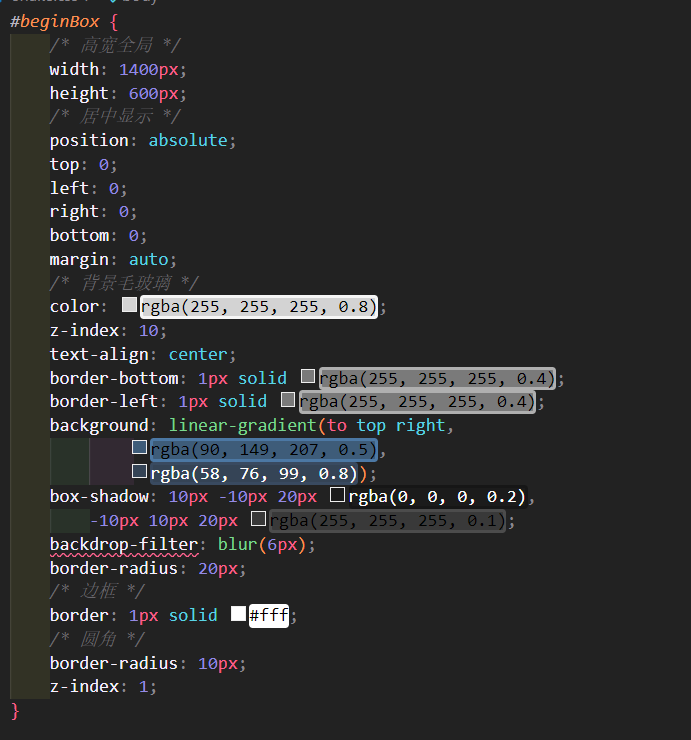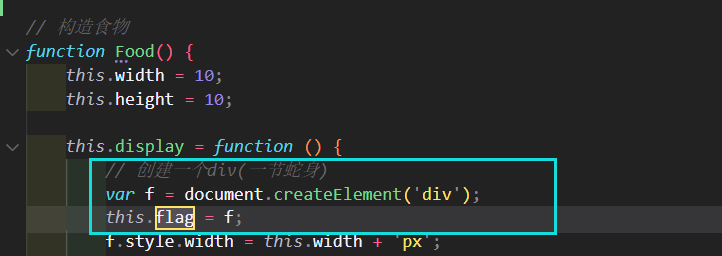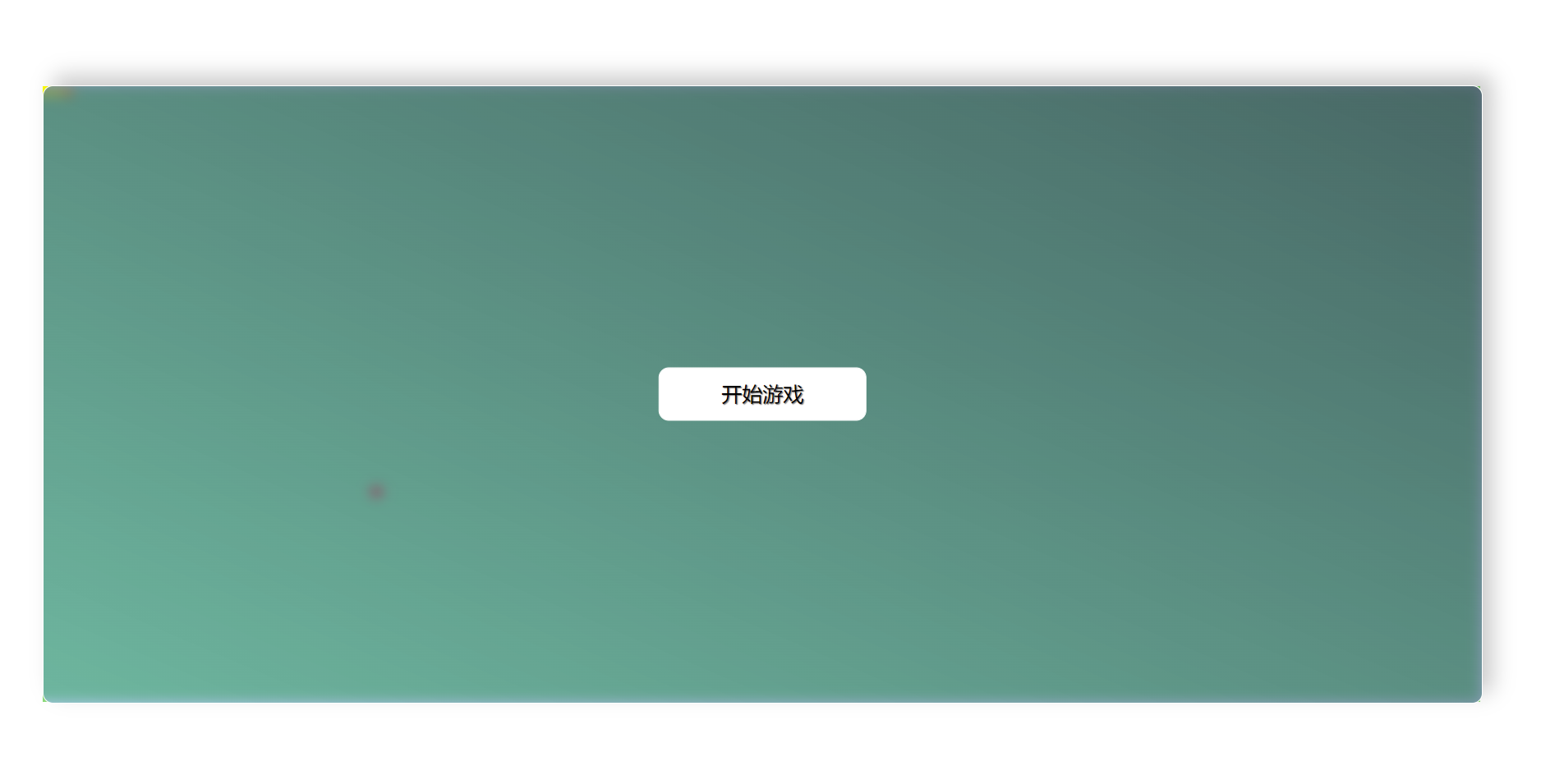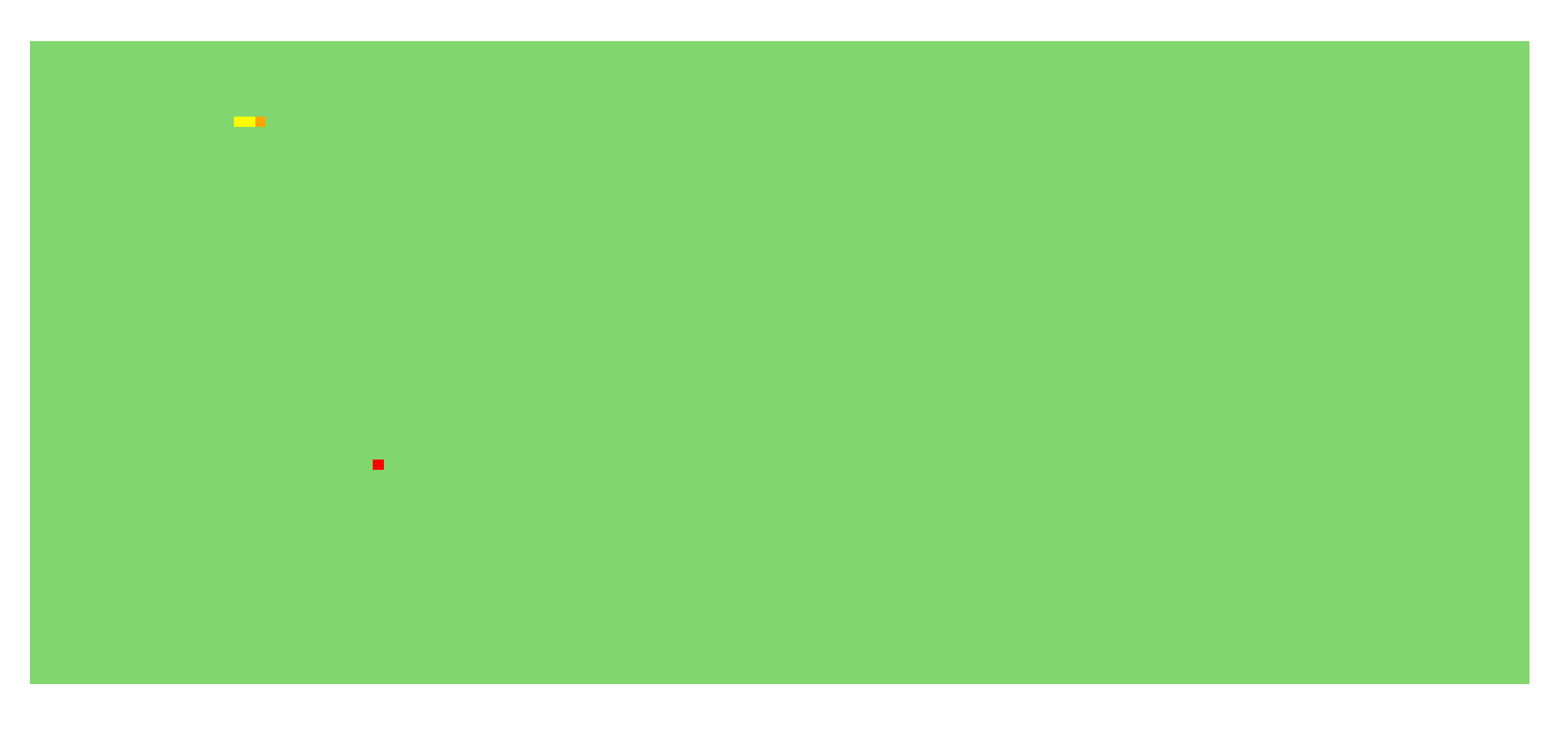# 用原生JavaScript寫一個貪吃蛇``                                開始遊戲                            ``

`beginBox`是開始遊戲的界面，我再這個盒子裡面實現瞭毛玻璃遮罩，還不錯。``// 蛇的速度，即計時器的間隔時間var SnakeTime = 200;// 蛇的身體var map = document.getElementById('map');``

``// 設置蛇的寬、高、默認走的方向    this.width = 10;    this.height = 10;    this.direction = 'right';``

``this.body = [    { x: 2, y: 0 },   // 蛇頭，第一個點    { x: 1, y: 0 },   // 蛇脖子，第二個點    { x: 0, y: 0 }    // 蛇尾，第三個點];``

``// 顯示蛇    this.display = function () {        // 創建蛇        for (var i = 0; i < this.body.length; i++) {            if (this.body[i].x != null) {                   // 當吃到食物時，x==null，不能新建，不然會在0，0處新建一個                var s = document.createElement('div');                // 將節點保存到狀態中，以便於後面刪除                this.body[i].flag = s;                // 設置寬高                s.style.width = this.width + 'px';                s.style.height = this.height + 'px';                //設置顏色                s.style.backgroundColor = 'yellow';                // 設置位置                s.style.position = 'absolute';                s.style.left = this.body[i].x * this.width + 'px';                s.style.top = this.body[i].y * this.height + 'px';                // 添加進去                map.appendChild(s);            }        }        //設置蛇頭的顏色        this.body.flag.style.backgroundColor = 'orange';    };``

``this.run = function () {        // 後一個元素到前一個元素的位置        for (var i = this.body.length - 1; i > 0; i--) {            this.body[i].x = this.body[i - 1].x;            this.body[i].y = this.body[i - 1].y;        }        // 根據方向處理蛇頭        switch (this.direction) {            case "left":                this.body.x -= 1;                break;            case "right":                this.body.x += 1;                break;            case "up":                this.body.y -= 1;                break;            case "down":                this.body.y += 1;                break;        }        // 判斷是否出界,根據蛇頭判斷        if (this.body.x  150 || this.body.y  60) {            clearInterval(timer);              // 清除定時器            alert("出界啦，遊戲結束！");            document.getElementById('beginBox').style.display = 'block';            // 刪除舊的            for (var i = 0; i < this.body.length; i++) {                if (this.body[i].flag != null) {                       // 如果剛吃完就死掉，會加一個值為null的                    map.removeChild(this.body[i].flag);                }            }            this.body = [   // 回到初始狀態，                { x: 2, y: 0 },                { x: 1, y: 0 },                { x: 0, y: 0 }            ];            this.direction = 'right';            this.display();   // 顯示初始狀態            return false;   // 結束        }        // 判斷蛇頭吃到食物，xy坐標重合，        if (this.body.x == food.x && this.body.y == food.y) {            // 蛇加一節，因為根據最後節點定，下面display時，會自動賦值的            this.body.push({ x: null, y: null, flag: null });            // 獲取蛇的長度            var len = this.body.length;            // 根據蛇的長度，設置定時器頻率SnakeTime            SnakeTime = SnakeTime - (len - 3) * 5;            // SnakeTime最低不能小於40            if (SnakeTime < 40) {                SnakeTime = 40;            }            refresh();            // 清除食物,重新生成食物            map.removeChild(food.flag);            food.display();        }        // 吃到自己死亡，從第五個開始與頭判斷，因為前四個永遠撞不到        for (var i = 4; i < this.body.length; i++) {            if (this.body.x == this.body[i].x && this.body.y == this.body[i].y) {                clearInterval(timer);   // 清除定時器，                alert("你咬到瞭自己，遊戲結束！");                // 顯示id為beginBox的毛玻璃遮罩盒子                document.getElementById('beginBox').style.display = 'block';                // 刪除舊的                for (var i = 0; i < this.body.length; i++) {                    if (this.body[i].flag != null) {                           // 如果剛吃完就死掉，會加一個值為null的                        map.removeChild(this.body[i].flag);                    }                }                this.body = [   // 回到初始狀態，                    { x: 2, y: 0 },                    { x: 1, y: 0 },                    { x: 0, y: 0 }                ];                this.direction = 'right';                this.display();   // 顯示初始狀態                return false;   // 結束            }        }        // 先刪掉初始的蛇，在顯示新蛇        for (var i = 0; i < this.body.length; i++) {            if (this.body[i].flag != null) {                   // 當吃到食物時，flag是等於null，且不能刪除                map.removeChild(this.body[i].flag);            }        }        // 重新顯示蛇        this.display();    }}``

``// 後一個元素到前一個元素的位置for (var i = this.body.length - 1; i > 0; i--) {      this.body[i].x = this.body[i - 1].x;      this.body[i].y = this.body[i - 1].y;}``

``// 根據方向處理蛇頭        switch (this.direction) {            case "left":                this.body.x -= 1;                break;            case "right":                this.body.x += 1;                break;            case "up":                this.body.y -= 1;                break;            case "down":                this.body.y += 1;                break;        }``

``// 判斷是否出界,根據蛇頭判斷        if (this.body.x  150 || this.body.y  60) {            clearInterval(timer);              // 清除定時器            alert("出界啦，遊戲結束！");            document.getElementById('beginBox').style.display = 'block';            // 刪除舊的            for (var i = 0; i < this.body.length; i++) {                if (this.body[i].flag != null) {                       // 如果剛吃完就死掉，會加一個值為null的                    map.removeChild(this.body[i].flag);                }            }            this.body = [   // 回到初始狀態，                { x: 2, y: 0 },                { x: 1, y: 0 },                { x: 0, y: 0 }            ];            this.direction = 'right';            this.display();   // 顯示初始狀態            return false;   // 結束        }``

``        // 判斷蛇頭吃到食物，xy坐標重合，        if (this.body.x == food.x && this.body.y == food.y) {            // 蛇加一節，因為根據最後節點定，下面display時，會自動賦值的            this.body.push({ x: null, y: null, flag: null });            // 獲取蛇的長度            var len = this.body.length;            // 根據蛇的長度，設置定時器頻率SnakeTime            SnakeTime = SnakeTime - (len - 3) * 5;            // SnakeTime最低不能小於40            if (SnakeTime < 40) {                SnakeTime = 40;            }            refresh();            // 清除食物,重新生成食物            map.removeChild(food.flag);            food.display();        }````// 獲取蛇的長度            var len = this.body.length;            // 根據蛇的長度，設置定時器頻率SnakeTime            SnakeTime = SnakeTime - (len - 3) * 5;            // SnakeTime最低不能小於40            if (SnakeTime < 40) {                SnakeTime = 40;            }``

``// 構造食物function Food() {    this.width = 10;    this.height = 10;    this.display = function () {        // 創建一個div(一節蛇身)        var f = document.createElement('div');        this.flag = f;        f.style.width = this.width + 'px';        f.style.height = this.height + 'px';        f.style.background = 'red';        f.style.position = 'absolute';        this.x = Math.floor(Math.random() * 80);        this.y = Math.floor(Math.random() * 40);        f.style.left = this.x * this.width + 'px';        f.style.top = this.y * this.height + 'px';        map.appendChild(f);    }}``

`` map.appendChild(f);``

``var snake = new Snake();var food = new Food();// 初始化顯示snake.display();   food.display();``

``// 給body加按鍵事件，上下左右document.body.onkeydown = function (e) {    // 有事件對象就用事件對象，沒有就自己創建一個，兼容低版本瀏覽器    var ev = e || window.event;    switch (ev.keyCode) {        case 38:            if (snake.direction != 'down') {   // 不允許返回，向上的時候不能向下                snake.direction = "up";            }            break;        case 40:            if (snake.direction != "up") {                snake.direction = "down";            }            break;        case 37:            if (snake.direction != "right") {                snake.direction = "left";            }            break;        case 39:            if (snake.direction != "left") {                snake.direction = "right";            }            break;        // 兼容WASD鍵            case 87:            if (snake.direction != "down") {                snake.direction = "up";            }            break;        case 83:            if (snake.direction != "up") {                snake.direction = "down";            }            break;        case 65:            if (snake.direction != "right") {                snake.direction = "left";            }            break;        case 68:            if (snake.direction != "left") {                snake.direction = "right";            }            break;    }    };``

``// 獲取開始按鈕var btn = document.getElementById('begin');// 點擊開始遊戲事件btn.onclick = function () {    // 開始按鈕毛玻璃幕佈    var parent = this.parentNode;    // 隱藏開始按鈕    parent.style.display = 'none';    // 獲取定時器時間    let time = SnakeTime;    timer = setInterval(function () {        snake.run();    }, time);}``

``    timer = setInterval(function () {        snake.run();    }, time);``

``// 定義刷新定時器方法function refresh() {    // 停止定時器    clearInterval(timer);    // 刷新定時器    timer = setInterval(function () {        snake.run();        console.log(SnakeTime);    }, SnakeTime);}```Github`：JanYork/Snake

`Gitee`：janyork/Snake